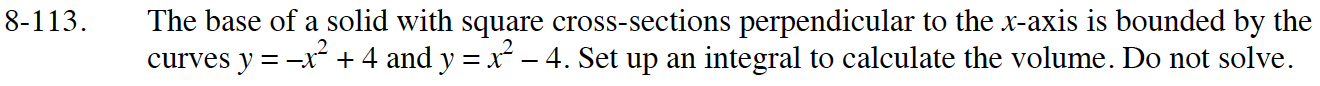### Home > APCALC > Chapter 8 > Lesson 8.3.2 > Problem8-113

8-113.

The base of a solid with square cross-sections perpendicular to the x-axis is bounded by the curves $y = -x^2 + 4$ and $y = x^2 - 4$. Set up an integral to calculate the volume. Do not solve. 6-113 HW eTool (Desmos). Homework Help ✎area of a $\text{square} = \text{(side)}^2$

Imagine a square that is built on the line connecting the "top" and "bottom" functions.
Write an expression for the length of the side of the square. Use symmetry to simplify the expression.

$\text{volume by cross-sections }= \int_{x=a}^{x=b}(A(x))dx\text{ so }\text{volume by cross-sections }= \int_{x=a}^{x=b}(\text{side})^{2}dx$

$\text{Think about this: volume}\neq 2\int_{-2}^{2}(x^{2}+4)^{2}dx.\text{ Why not?}$

Use the eTool below to help solve the problem.
Click on the link to the right to view the full version of the eTool. Calc 8-113 HW eTool